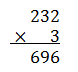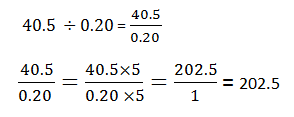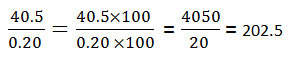# Multiplication And Division Of Decimals

Decimals are numbers with a decimal point (e.g.2.35). They represent the fraction of something and ten is the base of the decimal number system. A decimal notation or point differentiates an integer part from fractional part (e.g. 2.35 = 2 + 7/20).  Like whole numbers, decimals can also be added, subtracted, multiplied and divided. As compared to addition and subtraction of decimal numbers multiplication and division of decimals are much easy task. Both multiplication and division frequently connected in numerous daily computations. Even though concepts are confusing, it’s easy to solve them. Let’s go through multiplication and division of decimal fractions one by one.

## Multiplication of Decimals

When we multiply 10 by 2 it is similar to the addition of 10 two times:  10 x 10. This is same in the case of decimals as well. For example: 0.33 x 2= 0.33 + 0.33 + 0.33. Multiplication of decimal numbers is similar to a multiplication of whole numbers. Steps for multiplication of decimals are given below with an example.

Consider multiplication of two numbers, 2.32 and 3 for example.

Step 1: Take the count of the total number of places (digits) to the right of the decimal point in both numbers.

Here, in 2.32 there are two digits to the right of the decimal point and 3 is a whole number with no decimal point. Therefore, the total number of digits to the right of the decimal is 2.

Step 2: Now forget about the decimal point and just multiply the numbers without the decimal point.Step 3: After multiplication, put the decimal point in answer 2 places (step 1) from the right i.e. answer (2.32 x 3) will be 6.96.

Simply, just multiply the decimal numbers without decimal points and then give decimal point in the answer as many places same as the total number of places right to the decimal points in both numbers.

## Division of Decimals

If you divide decimal numbers as it is with a decimal point is quite confusing and difficult. We can use the same trick we used in the multiplication of decimals i.e. remove the decimal points and divide the numbers like whole numbers.

Let’s divide 40.5 by .20. Methods to divide these decimal numbers are as follows:

Method 1:  Convert the decimal numbers into whole numbers by multiplying both numerator and denominator by the same number. The denominator must be always a whole number.(Multiply both the numerator and denominator by 5)

Method 2:  Alternatively, one can convert decimal numbers into whole numbers by multiplying with numbers having powers of 10 (10, 100, 1000, etc.).

• Consider the denominator, count the number of places (digits) right to the decimal point.

Here, the denominator is 0.20 and the number of digits right to the decimal point is 2.

40.5 ÷ 0.20 = 40.5 / 0.20

• Take the power of 10 the same as the number of digits right to the decimal point e. 102 = 100
• Multiply both numerator and denominator by 100.### Multiplication and Division of Decimal Numbers by 10, 100 and 1000

As we know decimals are nothing but another form of expressing fractions having 10 as its base. We can express above examples as a fraction and can multiply and divide it as a fraction. Multiplication and division of decimals by numbers that have powers of 10 is easier than that by a whole number. Rules for multiplication and division of decimal numbers by 10, 100 and 1000:

 Arithmetic Operation Rule Example Multiply by 10 (101) the number will move one place value to the left 5.63 x 10 =56.3 Multiply by 100  (102) the number will move two places value to the left 5.63 x 100 =563 Multiply by 1000 (103) the number will move three places value to the left 5.63 x 1000 =5630 Divide by 10 (101) the number will move one place value to the right 56.3 ÷  10 =5.63 Divide by 100 (102) the number will move two places value to the right 56.3 ÷  100 =0.563 Divide by 1000 (103) the number will move three places value to the right 56.3 ÷  1000 =0.0563

To solve more problems on multiplication and division of decimals, download BYJU’S – The Learning App from Google Play Store and watch interactive videos.